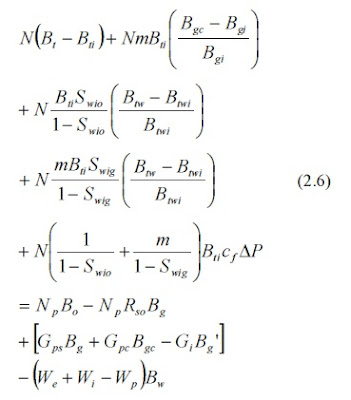# OIL RESERVOIR MATERIAL BALANCE

The general material balance equation for an oil reservoir is the Schilthuis material balance equation expressed in a form given by Guerrero:This equation is derived by conserving volume and is referred to as the volumetric material balance by Dake . The physical significance of the terms in Eq. (2.6) can be displayed by first defining the terms:
Substituting Eq. (2.7) in Eq. (2.6) gives the general material balance equation in the form:

The terms in Eq. (2.8) have a physical significance. The terms on the right hand side of Eq. (2.8) represent fluid production and injection, while the terms on the left hand side represent volume changes.

Equation (2.6) is considered a general material balance equation because it can be applied to an oil reservoir with a gas cap and an aquifer. The derivation of the material balance equation is based on several assumptions: the system is in pressure equilibrium; the system is isothermal; available fluid property data are representative of reservoir fluids; production data is reliable; and gravity segregation of phases can be neglected.